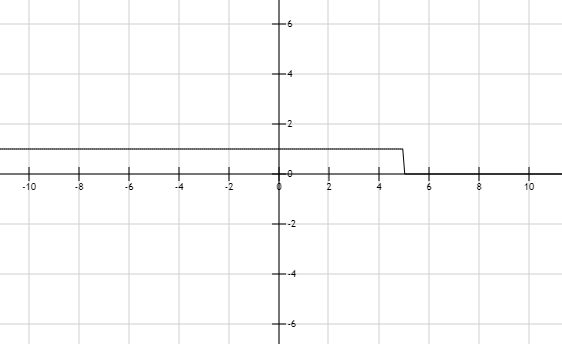# The solution of given linear inequality equation and plot a graph for it.### Precalculus: Mathematics for Calcu...

6th Edition
Stewart + 5 others
Publisher: Cengage Learning
ISBN: 9780840068071### Precalculus: Mathematics for Calcu...

6th Edition
Stewart + 5 others
Publisher: Cengage Learning
ISBN: 9780840068071

#### Solutions

Chapter 1.7, Problem 26E
To determine

## The solution of given linear inequality equation and plot a graph for it.

Expert Solution

x5

### Explanation of Solution

Given:

Linear inequality equation

2(7x3)12x+16

Calculation,

Linear inequality equation,

2(7x3)12x+1614x612x+1614x12x1662x10x102x5

Now, graph of 2(7x3)12x+16 ,Conclusion:

Hence, the values of x is less than equal to the 5.

### Have a homework question?

Subscribe to bartleby learn! Ask subject matter experts 30 homework questions each month. Plus, you’ll have access to millions of step-by-step textbook answers!